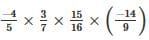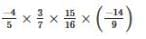# Test: Rational Numbers

## 10 Questions MCQ Test NCERT Mathematics for CAT Preparation | Test: Rational Numbers

Description
Attempt Test: Rational Numbers | 10 questions in 10 minutes | Mock test for Class 7 preparation | Free important questions MCQ to study NCERT Mathematics for CAT Preparation for Class 7 Exam | Download free PDF with solutions
QUESTION: 1

### Associative property is not followed in _____

Solution:

In general associative properties also holds true addition as well as multiplication for all four options as well as but not true for subtraction as well as division.

If we add zero in the natural number then we also get set of numbers which is called as the whole number.

QUESTION: 2

### ____ is the identity for the addition of rational numbers.

Solution:

Additive identity means that to any number if this identity is added, the number remains the same. So additive identity is 0 which means 0 added to any no. does not affect it.

QUESTION: 3

### ____ is the multiplicative identity for rational numbers.

Solution:

1 is the multiplicative identity which means that identity of the number remains intact if it is multiplied by 1

QUESTION: 4

Which of the following is the reciprocal of the reciprocal of a rational number?

Solution:

Let we have a rational number having numerator as 2 and denominator as 3. The rational form is given by :

2/3

Reciprocal of a number is such that the numerator becomes denominator and the denominator becomes numerator. So, the reciprocal of the above rational no will be :

3/2

The reciprocal of the reciprocal of a rational number will be again  2/3. Hence, the reciprocal of the reciprocal of a rational number is the number itself.

QUESTION: 5

The product of two numbers is -20/9. If one of the numbers is 4, find the other.

Solution:

We have x * 4 = -20/9
x = -20 / 9* 4= -5/9

QUESTION: 6

The numbers ________ and ________ are their own reciprocals.

Solution:

Reciprocal of 1 and -1 are their own reciprocals.

1 = 1/1 and its reciprocal is 1/1 i.e. 1. ( Number itself)

-1= -1/1

Its reciprocal= 1/-1= -1 (number itself)

QUESTION: 7

The reciprocal of – 5 is ________

Solution:
QUESTION: 8

Reciprocal of 1/x, where x ≠ 0 is ________.

Solution:

Reciprocal is the inverse of the number, meaning, the numerator becomes denominator and denominator becomes numerator.so 1/x become x/1

QUESTION: 9

The product of two rational numbers is always a _____.

Solution:
QUESTION: 10

simplify :Solution:⇒ -1 x 1 x 3/4 x -2/3

⇒ -1 x -1/2

⇒ 1/2Use Code STAYHOME200 and get INR 200 additional OFF Use Coupon Code